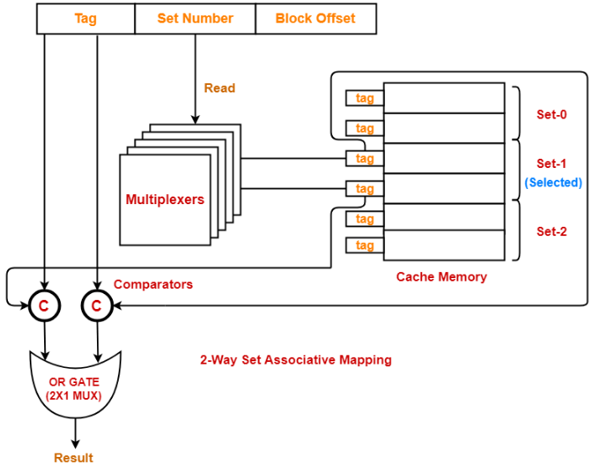# Working and implementation of Set-associative Mapped Cache

In this article, we are going to learn about the working of Set-associative mapped Cache with a diagram. After we will see the steps of implementation and a problem based on Set-associative Cache.
Submitted by Monika Jha, on November 19, 2019

Prerequisites: Memory mapping and its types

## Set-associative Cache Mapping

In this mapping technique, blocks of cache are grouped to form a set and a block of main memory can go into any block of a specific set.

## Working of Set-associative Cache

• CPU generates a memory request then the set number field of the address is used to access the particular set of the cache.
• The tag field of the processor address is then compared with the tags of all K lines within that set.
• If these two tags do not match the tag of any cache line, a cache miss occurs.
• If these two tags match, a cache hit occurs.
• In case of a cache miss, the required word has to be brought from the main memory.
• If the cache is full, a replacement is made according to the employed replacement policy.

A diagram to show the implementation of set-associative mapped cache as follows,Steps to implement set-associative cache mapping

Step 1:

• Each multiplexer scans the set number from the generated physical address using its select lines in parallel.
• The number of select lines of each multiplexer should be S to read the set number of S bits.

Step 2:

• After reading the set number, every multiplexer goes to the corresponding set within the cache memory.
• After that, every multiplexer goes to the lines of that set using its input lines in parallel.
• Number of input lines of every multiplexer = Number of lines in one set

Step 3:

• Each multiplexer outputs the tag but it's elect from the lines of elect set to the comparators using its output line.
• The number of output lines in every multiplexer = one.
• Each electronic device selects the tag bit that it's been designed and outputs on the output line as multiplexer is designed to read the tag little bit of specific line at a specific location.
• So, the tags as a whole are transmitted to the comparators for comparison in parallel.

Step 4:

• Comparators compare the tags coming back from the multiplexers with the tag of the generated address.
• This comparison takes place in parallel.
• If there are k lines in one set (thus k tags), then the number of comparators required = k.
• Size of each comparator = Number of bits in the tag.
• The output result of each comparator is fed as an input to an OR Gate.
• OR Gate is usually implemented using 2 x 1 multiplexer.
• If the output of OR Gate is 0, a cache miss occurs and if the output is 1 then a cache hit occurs.

### Hit latency

The time taken to search out whether or not the specified word is available within the Cache Memory or not is termed as hit latency.

For set-associative cache mapping: Hit latency = Multiplexer latency + Comparator latency + OR Gate latency.

### Problem based on direct mapped cache

If there is a 4-way set associative mapped cache with block size 4 KB and the size of main memory is 16 GB and also 10 bits in the tag. Find,

1. Size of cache memory
2. Tag directory size

Solution:

```    We are considering that the memory is byte addressable.
Block size or Frame size or Line size = 4 KB

Number of Bits in Physical Address-
Size of main memory
= 16 GB
= 234 bytes
Hence, num of bits in physical address = 34 bits

Number of Bits in Block Offset-
Block size
= 4 KB
= 212 bytes
Hence, Number of bits in block offset = 12 bits

Number of Bits in Set Number
= Num of bits in physical address – (Num of bits in tag + Num of bits in block offset)
= 34 bits – (10 bits + 12 bits)
= 34 bits – 22 bits
= 12 bits
Hence, Number of bits in set number = 12 bits

Number of Sets in Cache-
Total num of bits in set number = 12 bits
Hence, the total number of sets in cache = 212 sets
And each set consists of 4 lines

Hence, total number of lines in cache = Total number of sets in cache x Number of lines in each set
= 212 x 4 lines
= 214 lines

Size of cache memory = Total number of lines in cache x size of line
= 214 x 4 KB
= 216 x 22KB
= 64 MB
So, Size of cache memory = 64 MB

Tag directory size = Number of tags x size of tag
= the total num of lines in cache x total number of bits in tag
= 214 x 10 bits
= 163840 bits
= 20480 bytes
= 20 KB
Hence, size of tag directory = 20 KB
```

References:

What's New

Top Interview Coding Problems/Challenges!

Languages: » C » C++ » C++ STL » Java » Data Structure » C#.Net » Android » Kotlin » SQL
Web Technologies: » PHP » Python » JavaScript » CSS » Ajax » Node.js » Web programming/HTML
Solved programs: » C » C++ » DS » Java » C#
Aptitude que. & ans.: » C » C++ » Java » DBMS
Interview que. & ans.: » C » Embedded C » Java » SEO » HR
CS Subjects: » CS Basics » O.S. » Networks » DBMS » Embedded Systems » Cloud Computing
» Machine learning » CS Organizations » Linux » DOS
More: » Articles » Puzzles » News/Updates